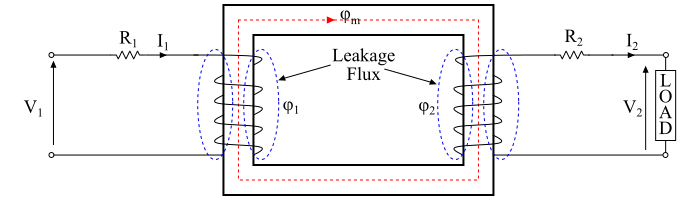# What is a Practical Transformer?

A practical transformer is the one which has following properties −

• The primary and secondary windings have finite resistance.
• There is a leakage flux, i.e., whole of the flux is not confined to the magnetic circuit.
• The magnetic core has finite permeability, thus a considerable amount of mmf is require to establish flux in the core.
• There are losses in the transformer due to winding resistances, hysteresis and eddy currents. Therefore, the efficiency of a practical transformer is less than 100 %.

The figure shows a typical practical transformer, which possess all the characteristics that are described above.## Winding Resistances

As the windings of a transformer are made up of copper conductors. Therefore, both the primary and secondary windings will have winding resistances, which produce the copper loss or I2R loss in the transformer. The primary winding resistance R1 and the secondary winding resistance R2 act in series with the respective windings (see the figure).

## Iron Losses or Core Losses

The core of the transformer is subjected to the alternating magnetic flux, hence there occurs eddy current loss and hysteresis loss in the core. The hysteresis loss and eddy current loss together are known as iron losses or core losses. The iron losses of the transformer depend upon the supply frequency, maximum flux density in the core, volume of the core and thickness of the laminations etc. In a practical transformer, the magnitude of iron losses is very small.

## Magnetic Leakage or Leakage Flux

As the current through the primary winding produces flux. The flux ϕm which links both the windings is the useful flux and is known as mutual flux. Although, a part of the flux (ϕ1) produced by the primary current does not link with the secondary winding. When load is connected across the secondary winding, a current flows through it and produces a flux (ϕ2), which links only with the secondary winding. Thus, the fluxes such as ϕ1 or ϕ2 which links only one winding is known as leakage flux.

The leakage flux paths are mainly through the air which has very high reluctance. Therefore, the effect of primary leakage flux (ϕ1) is to introduce an inductive reactance (X1) in series with the primary winding. Similarly, the secondary leakage flux (ϕ) introduces an inductive reactance (X2) in series with the secondary winding (see the figure).However, the leakage flux in a practical transformer is very small (about 5% of ϕm), yet it cannot be ignored. Because, the leakage flux paths are through the air, which has very high reluctance. As a result, it requires considerable mmf.

## Finite Permeability of Core Material

In general, the practical transformers have a core made up of silicon steel, which has a specific relative permeability (μr). Hence, the core saturates at a certain value of magnetic flux density. Therefore, the core of a practical transformer has finite permeability and hence possess reluctance in the path of magnetic flux.